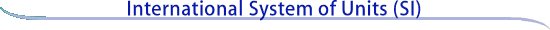Return to Units home page Units Topics: Base Derived Prefixes Non-SI Rules Background Units Bibliography Constants,Units &Uncertaintyhome pageBecome familiar with the seven defining constants of the SI. The following seven SI base unit definitions are based on the BIPM SI Brochure (9th Edition).

Definitions of the SI base units

 Unit of length meter The meter, symbol m, is the SI unit of length. It is defined by taking the fixed numerical value of the speed of light in vacuum c to be 299 792 458 when expressed in the unit m s-1, where the second is defined in terms of ΔνCs. Unit of mass kilogram The kilogram, symbol kg, is the SI unit of mass. It is defined by taking the fixed numerical value of the Planck constant h to be 6.626 070 15 × 10-34 when expressed in the unit J s, which is equal to kg m2 s-1, where the meter and the second are defined in terms of c and ΔνCs. Unit of time second The second, symbol s, is the SI unit of time. It is defined by taking the fixed numerical value of the cesium frequency ΔνCs, the unperturbed ground-state hyperfine transition frequency of the cesium 133 atom, to be 9 192 631 770 when expressed in the unit Hz, which is equal to s-1. Unit of electric current ampere The ampere, symbol A, is the SI unit of electric current. It is defined by taking the fixed numerical value of the elementary charge e to be 1.602 176 634 x 10-19 when expressed in the unit C, which is equal to A s, where the second is defined in terms of ΔνCs. Unit of thermodynamic   temperature kelvin The kelvin, symbol K, is the SI unit of thermodynamic temperature. It is defined by taking the fixed numerical value of the Boltzmann constant k to be 1.380 649 x 10-23 when expressed in the unit J K-1, which is equal to kg m2 s-2 K-1, where the kilogram, meter and second are defined in terms of h, c and ΔνCs. Unit of amount of substance mole The mole, symbol mol, is the SI unit of amount of substance. One mole contains exactly 6.022 140 76 x 1023 elementary entities. This number is the fixed numerical value of the Avogadro constant, NA, when expressed in the unit mol-1 and is called the Avogadro number. The amount of substance, symbol n, of a system is a measure of the number of specified elementary entities. An elementary entity may be an atom, a molecule, an ion, an electron, any other particle or specified group of particles. Unit of luminous intensity candela The candela, symbol cd, is the SI unit of luminous intensity in a given direction. It is defined by taking the fixed numerical value of the luminous efficacy of monochromatic radiation of frequency 540 x 1012 Hz, Kcd, to be 683 when expressed in the unit lm W-1, which is equal to cd sr W-1, or cd sr kg-1 m-2 s3, where the kilogram, meter and second are defined in terms of h, c and ΔνCs.

Go to SI Units Background or SI base units

Online: March 1998   -   Last update: June 2019